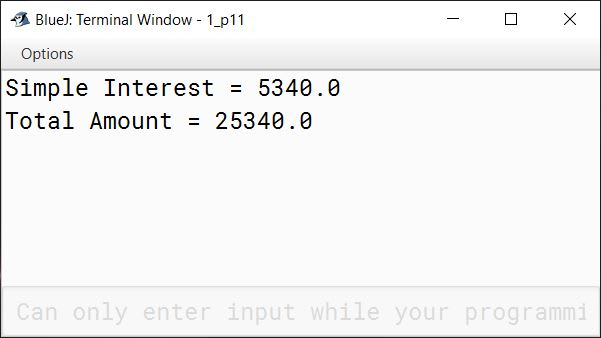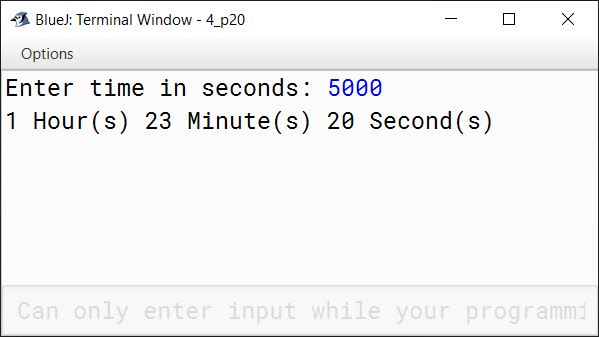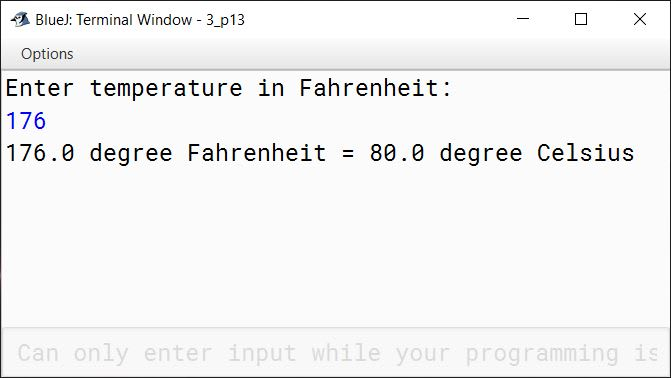# Input in Java

## Multiple Choice Questions

#### Question 1

1. //
2. /* */
3. \\
4. Both A and B ✓

#### Question 2

Which keyword do you use to include a class in your program?

1. import ✓
2. export
3. include
4. impart

#### Question 3

Default delimiter used in the Scanner class is ...........

1. Comma
2. Whitespace ✓
3. Colon
4. There is no default delimiter.

#### Question 4

Which package would you import to display the date and time?

1. java.util ✓
2. java.Date
3. java.io
4. java.lang

#### Question 5

Which package would you import for the Scanner class?

1. java.util.* ✓
2. java.awt.*
3. java.io.*
4. java.lang.*

#### Question 6

Errors occur in a program when ...........

1. Syntax of the programming language is not followed.
2. The program does not run properly or does not execute at all.
3. The program produces an incorrect result.
4. All of the above ✓

## Assignment Questions

#### Question 1

What is a java package? Give an example.

A package is a named collection of Java classes that are grouped on the basis of their functionality. For example, java.util.

#### Question 2

Explain the use of import statement with an example.

import statement is used to import built-in and user-defined packages and classes into our Java program. For example, we can import all the classes present in the java.util package into our program using the below statement:

`import java.util.*;`

#### Question 3

Explain the statement, "a well-documented code is as important as the correctly working code".

Writing fully commented code is a good programming style. The primary purpose of comments is to document the code so that even a layman can understand the purpose of the written code. Hence, a well-documented code is as important as the correctly working code.

#### Question 4

How can you write single line comments in Java?

Single line comments in Java can be written in the below two ways:

1. Using Single line comments style — Single line comment begin with a double slash (//) and continues till the end of the line. For example:
`// Single line comment example`
1. Using Multi line comments style — In this style, comments start with the initiating slash-asterisk (/*) and ends with the terminating asterisk-slash(*/). For example:
`/* Single line comment using multi line comment style */`

#### Question 5

Explain data input technique in a program using the Scanner class.

The data entered by the user through the keyboard is provided to our Java program with the help of standard input stream that is specified as System.in in the program. We connect our Scanner class to this stream while creating its object. The input received by Scanner class is broken into tokens using a delimiter pattern. The default delimiter is whitespace. Scanner class provides various next methods to read these tokens from the stream. The different next methods provided by Scanner class are next(), nextShort(), nextInt(), nextLong(), nextFloat(), nextDouble(), nextLine(), next().charAt(0).

#### Question 6

Distinguish between the following:

i. next() and nextLine()

next()nextLine()
It reads the input only till the next complete token until the delimiter is encountered.It reads the input till the end of line so it can read a full sentence including spaces.
It places the cursor in the same line after reading the input.It places the cursor in the next line after reading the input.

ii. next() and next().charAt(0)

next()next().charAt(0)
It reads the input only till the next complete token until the delimiter is encountered.It reads the complete token and then the first character is returned using the charAt(0) method.
It returns the read data as a String value.It returns the read data as a char value.

iii. next() and hasNext()

next()hasNext()
It reads the input till the next complete token until the delimiter is encountered.It checks if the Scanner has another token in its input.
It returns a String value.It returns a boolean value.

iv. hasNext() and hasNextLine()

hasNext()hasNextLine()
Returns true if the Scanner object has another token in its input.Returns true if there is another line in the input of the Scanner object.

#### Question 7

What are delimiters? Which is the default delimiter used in the Scanner class?

A delimiter is a sequence of one or more characters that separates two tokens. The default delimiter is a whitespace.

#### Question 8

What are errors in a program?

Errors are mistakes in a program that prevents it from its normal working. They are often referred to as bugs. Errors are classified into three categories — Syntax Errors, Runtime Errors and Logical Errors.

#### Question 9

Explain the following terms, giving an example of each.

i. Syntax error

Syntax Errors are the errors that occur when we violate the rules of writing the statements of the programming language. These errors are reported by the compiler and they prevent the program from executing. For example, the following statement will give an error as we missed terminating it with a semicolon:

`int sum`

ii. Runtime error

Errors that occur during the execution of the program primarily due to the state of the program which can only be resolved at runtime are called Run Time errors.
Consider the below example:

```import java.util.Scanner;

class RunTimeError
{
public static void main(String args[]) {
Scanner in = new Scanner(System.in);
System.out.print("Enter a number: ");
int n = in.nextInt();
int result = 100 / n;
System.out.println("Result = " + result);
}
}```

This program will work fine for all non-zero values of n entered by the user. When the user enters zero, a run-time error will occur as the program is trying to perform an illegal mathematical operation of division by 0. When we are compiling the program, we cannot say if division by 0 error will occur or not. It entirely depends on the state of the program at run-time.

iii. Logical error

When a program compiles and runs without errors but produces an incorrect result, this type of error is known as logical error. It is caused by a mistake in the program logic which is often due to human error. Logical errors are not caught by the compiler. Consider the below example for finding the perimeter of a rectangle with length 2 and breadth 4:

```class RectanglePerimeter
{
public static void main(String args[]) {
int l = 2, b = 4;
double perimeter = 2 * (l * b);    //This line has a logical error
System.out.println("Perimeter = " + perimeter);
}
}```
##### Output
``````Perimeter = 16
``````

The program gives an incorrect perimeter value of 16 as the output due to a logical error. The correct perimeter is 12. We have written the formula of calculating perimeter incorrectly in the line `double perimeter = 2 * (l * b);`. The correct line should be `double perimeter = 2 * (l + b);`.

#### Question 10

A program has compiled successfully without any errors. Does this mean the program is error free? Explain.

Successful compilation of the program only ensures that there are no syntax errors. Runtime and logical errors can still be there in the program so we cannot say that the program is error free. Only after comprehensive testing of the program, we can say that the program is error free.

#### Question 11

Write a program in Java that uses 'input using initialisation' to calculate the Simple Interest and the Total Amount with the given values:

i. Principle Amount = Rs. 20000
ii. Rate = 8.9%
iii. Time = 3 years

```public class KboatInterest
{
public static void main(String args[]) {
int p = 20000;
double r = 8.9;
int t = 3;
double si = p * r * t / 100.0;
double amt = p + si;
System.out.println("Simple Interest = " + si);
System.out.println("Total Amount = " + amt);
}
}```
##### Output#### Question 12

Write a program in Java that accepts the seconds as input and converts them into the corresponding number of hours, minutes and seconds. A sample output is shown below:

``````Enter Total Seconds:
5000
1 Hour(s) 23 Minute(s) 20 Second(s)

``````

```import java.util.Scanner;

public class KboatTimeConvert
{
public static void main(String args[]) {
Scanner in = new Scanner(System.in);
System.out.print("Enter time in seconds: ");
long secs = in.nextLong();
long hrs = secs / 3600;
secs %= 3600;
long mins = secs / 60;
secs %= 60;
System.out.println(hrs + " Hour(s) " + mins
+ " Minute(s) " + secs + " Second(s)");
in.close();
}
}```
##### Output#### Question 13

Write a program in java to input the temperature in Fahrenheit, convert it into Celsius and display the value in the Terminal window. A sample output is displayed below:

``````Enter temperature in Fahrenheit
176
176.0 degree Fahrenheit = 80.0 degree Celsius
``````

```import java.util.Scanner;

public class KboatTemperature
{
public static void main(String args[]) {
Scanner in = new Scanner(System.in);
System.out.println("Enter temperature in Fahrenheit: ");
double f = in.nextDouble();
double c = (f - 32) * 5.0 / 9.0;
System.out.println(f + " degree Fahrenheit = " + c + " degree Celsius");
}
}```
##### Output